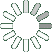Puzzle Command
Daily Puzzle
Number Logic #24
April 12, 2015Find a 6 digit number where:

The 1st digit is the number of 3s in the number. The 2nd digit is any number. The 3rd digit is an odd number. The 4th digit is equal to 5. The 5th digit is equal to 5. The 6th digit is the sum of the 1st and 4th digit.

All digits are higher than 2.

Puzzle Notes
6 digit numbers range from 100,000 to 999,999.
Enter in your solution below and click submit.